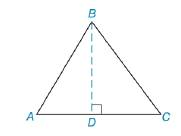Chapter 8.1, Problem 40EElementary Geometry For College St...

7th Edition
Alexander + 2 others
ISBN: 9781337614085

Solutions

Chapter
SectionElementary Geometry For College St...

7th Edition
Alexander + 2 others
ISBN: 9781337614085
Textbook Problem

Given: △ A B C , whose sides are 10 cm, 17 cm, and 21 cm. Find: a) BD, the length of the altitude to the 21-cm side b) The area of △ A B C , using the result from part (a)Exercises 39, 40

To determine

a)

To find:

The length of the altitude.

Explanation

Find the length of the altitude of the triangle is followed by the formula.

By Pythagorean theorem,

(BD)2+(DC)2=(BC)2

Calculation:

Given,

ABC, whose sides are 10 cm., 17 cm., and 21 cm., the length of the altitude BD to the 21-cm. side.

Let x be the length of DC and h be the length of the altitude BD.

For the right triangle BDC,

DC=x cm., BD=h cm., and BC=10 cm.

By Pythagorean theorem,

(BD)2+(DC)2=(BC)2

(h)2+(x)2=(10)2

h2+x2=100

Add (x2) on both sides,

h2+x2+(x2)=100+(x2)

h2+x2x2=100x2

h2=100x2

For the right triangle ADB,

AD=(21x) cm, BD=h cm, and AB=17 cm

To determine

b)

To find:

The area of ABC, using the result from part (a).

Still sussing out bartleby?

Check out a sample textbook solution.

See a sample solution

The Solution to Your Study Problems

Bartleby provides explanations to thousands of textbook problems written by our experts, many with advanced degrees!

Get Started

Find more solutions based on key concepts